# 计算传播与机器学习¶

[email protected]## 1、 监督式学习¶

• 这个算法由一个目标变量或结果变量（或因变量）组成。
• 这些变量由已知的一系列预示变量（自变量）预测而来。
• 利用这一系列变量，我们生成一个将输入值映射到期望输出值的函数。
• 这个训练过程会一直持续，直到模型在训练数据上获得期望的精确度。
• 监督式学习的例子有：回归、决策树、随机森林、K – 近邻算法、逻辑回归等。

## 2、非监督式学习¶

• 在这个算法中，没有任何目标变量或结果变量要预测或估计。
• 这个算法用在不同的组内聚类分析。
• 这种分析方式被广泛地用来细分客户，根据干预的方式分为不同的用户组。
• 非监督式学习的例子有：关联算法和 K–均值算法。

## 3、强化学习¶

• 这个算法训练机器进行决策。
• 它是这样工作的：机器被放在一个能让它通过反复试错来训练自己的环境中。
• 机器从过去的经验中进行学习，并且尝试利用了解最透彻的知识作出精确的商业判断。
• 强化学习的例子有马尔可夫决策过程。alphago

Chess. Here, the agent decides upon a series of moves depending on the state of the board (the environment), and the reward can be defined as win or lose at the end of the game:• 线性回归
• 逻辑回归
• 决策树
• SVM
• 朴素贝叶斯

• K最近邻算法
• K均值算法
• 随机森林算法
• 降维算法
• Gradient Boost 和 Adaboost 算法

# 使用sklearn做线性回归¶

[email protected]

# 线性回归¶

• 通常用于估计连续性变量的实际数值（房价、呼叫次数、总销售额等）。
• 通过拟合最佳直线来建立自变量X和因变量Y的关系。
• 这条最佳直线叫做回归线，并且用 $Y= \beta *X + C$ 这条线性等式来表示。
• 系数 $\beta$ 和 C 可以通过最小二乘法获得
In :
%matplotlib inline
import sklearn
from sklearn import datasets
from sklearn import linear_model
import matplotlib.pyplot as plt
from sklearn.metrics import classification_report
from sklearn.preprocessing import scale

In :
# boston data
boston = datasets.load_boston()
y = boston.target
X = boston.data

In :
' '.join(dir(boston))

Out:
'__class__ __contains__ __delattr__ __delitem__ __dict__ __dir__ __doc__ __eq__ __format__ __ge__ __getattr__ __getattribute__ __getitem__ __gt__ __hash__ __init__ __iter__ __le__ __len__ __lt__ __module__ __ne__ __new__ __reduce__ __reduce_ex__ __repr__ __setattr__ __setitem__ __setstate__ __sizeof__ __str__ __subclasshook__ __weakref__ clear copy fromkeys get items keys pop popitem setdefault update values'
In :
boston['feature_names']

Out:
array(['CRIM', 'ZN', 'INDUS', 'CHAS', 'NOX', 'RM', 'AGE', 'DIS', 'RAD',
'TAX', 'PTRATIO', 'B', 'LSTAT'],
dtype='<U7')
In :
import numpy as np
import statsmodels.api as sm
import statsmodels.formula.api as smf

# Fit regression model (using the natural log of one of the regressors)
results = smf.ols('boston.target ~ boston.data', data=boston).fit()

print(results.summary())

                            OLS Regression Results
==============================================================================
Dep. Variable:          boston.target   R-squared:                       0.741
Model:                            OLS   Adj. R-squared:                  0.734
Method:                 Least Squares   F-statistic:                     108.1
Date:                Sun, 29 Apr 2018   Prob (F-statistic):          6.95e-135
Time:                        15:12:28   Log-Likelihood:                -1498.8
No. Observations:                 506   AIC:                             3026.
Df Residuals:                     492   BIC:                             3085.
Df Model:                          13
Covariance Type:            nonrobust
===================================================================================
coef    std err          t      P>|t|      [95.0% Conf. Int.]
-----------------------------------------------------------------------------------
Intercept          36.4911      5.104      7.149      0.000        26.462    46.520
boston.data     -0.1072      0.033     -3.276      0.001        -0.171    -0.043
boston.data      0.0464      0.014      3.380      0.001         0.019     0.073
boston.data      0.0209      0.061      0.339      0.735        -0.100     0.142
boston.data      2.6886      0.862      3.120      0.002         0.996     4.381
boston.data    -17.7958      3.821     -4.658      0.000       -25.302   -10.289
boston.data      3.8048      0.418      9.102      0.000         2.983     4.626
boston.data      0.0008      0.013      0.057      0.955        -0.025     0.027
boston.data     -1.4758      0.199     -7.398      0.000        -1.868    -1.084
boston.data      0.3057      0.066      4.608      0.000         0.175     0.436
boston.data     -0.0123      0.004     -3.278      0.001        -0.020    -0.005
boston.data    -0.9535      0.131     -7.287      0.000        -1.211    -0.696
boston.data     0.0094      0.003      3.500      0.001         0.004     0.015
boston.data    -0.5255      0.051    -10.366      0.000        -0.625    -0.426
==============================================================================
Omnibus:                      178.029   Durbin-Watson:                   1.078
Prob(Omnibus):                  0.000   Jarque-Bera (JB):              782.015
Skew:                           1.521   Prob(JB):                    1.54e-170
Kurtosis:                       8.276   Cond. No.                     1.51e+04
==============================================================================

Warnings:
 Standard Errors assume that the covariance matrix of the errors is correctly specified.
 The condition number is large, 1.51e+04. This might indicate that there are
strong multicollinearity or other numerical problems.

In :
regr = linear_model.LinearRegression()
lm = regr.fit(boston.data, y)

In :
lm.intercept_, lm.coef_, lm.score(boston.data, y)

Out:
(36.491103280363603,
array([ -1.07170557e-01,   4.63952195e-02,   2.08602395e-02,
2.68856140e+00,  -1.77957587e+01,   3.80475246e+00,
7.51061703e-04,  -1.47575880e+00,   3.05655038e-01,
-1.23293463e-02,  -9.53463555e-01,   9.39251272e-03,
-5.25466633e-01]),
0.74060774286494269)
In :
predicted = regr.predict(boston.data)

In :
fig, ax = plt.subplots()
ax.scatter(y, predicted)
ax.plot([y.min(), y.max()], [y.min(), y.max()], 'k--', lw=4)
ax.set_xlabel('$Measured$', fontsize = 20)
ax.set_ylabel('$Predicted$', fontsize = 20)
plt.show()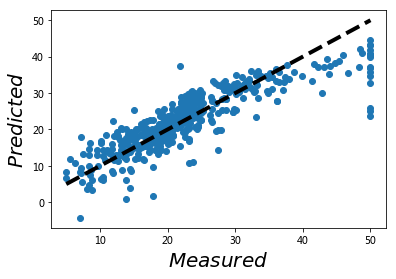## 训练集和测试集¶

In :
boston.data

Out:
array([[  6.32000000e-03,   1.80000000e+01,   2.31000000e+00, ...,
1.53000000e+01,   3.96900000e+02,   4.98000000e+00],
[  2.73100000e-02,   0.00000000e+00,   7.07000000e+00, ...,
1.78000000e+01,   3.96900000e+02,   9.14000000e+00],
[  2.72900000e-02,   0.00000000e+00,   7.07000000e+00, ...,
1.78000000e+01,   3.92830000e+02,   4.03000000e+00],
...,
[  6.07600000e-02,   0.00000000e+00,   1.19300000e+01, ...,
2.10000000e+01,   3.96900000e+02,   5.64000000e+00],
[  1.09590000e-01,   0.00000000e+00,   1.19300000e+01, ...,
2.10000000e+01,   3.93450000e+02,   6.48000000e+00],
[  4.74100000e-02,   0.00000000e+00,   1.19300000e+01, ...,
2.10000000e+01,   3.96900000e+02,   7.88000000e+00]])
In :
from sklearn.cross_validation import train_test_split
Xs_train, Xs_test, y_train, y_test = train_test_split(boston.data,
boston.target,
test_size=0.2,
random_state=42)

In :
regr = linear_model.LinearRegression()
lm = regr.fit(Xs_train, y_train)

In :
lm.intercept_, lm.coef_, lm.score(Xs_train, y_train)

Out:
(30.288948339369036,
array([ -1.12463481e-01,   3.00810168e-02,   4.07309919e-02,
2.78676719e+00,  -1.72406347e+01,   4.43248784e+00,
-6.23998173e-03,  -1.44848504e+00,   2.62113793e-01,
-1.06390978e-02,  -9.16398679e-01,   1.24516469e-02,
-5.09349120e-01]),
0.75088377867329148)
In :
predicted = regr.predict(Xs_test)

In :
fig, ax = plt.subplots()
ax.scatter(y_test, predicted)
ax.plot([y.min(), y.max()], [y.min(), y.max()], 'k--', lw=4)
ax.set_xlabel('$Measured$', fontsize = 20)
ax.set_ylabel('$Predicted$', fontsize = 20)
plt.show()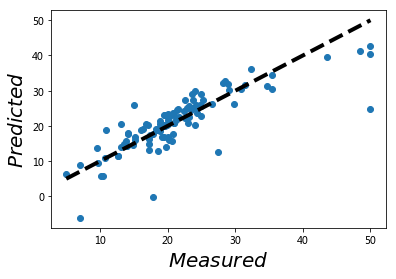# cross-validation¶

k-fold CV, the training set is split into k smaller sets (other approaches are described below, but generally follow the same principles). The following procedure is followed for each of the k “folds”:

• A model is trained using k-1 of the folds as training data;
• the resulting model is validated on the remaining part of the data (i.e., it is used as a test set to compute a performance measure such as accuracy).
In :
from sklearn.cross_validation import cross_val_score

regr = linear_model.LinearRegression()
scores = cross_val_score(regr, boston.data , boston.target, cv = 3)
scores.mean()

Out:
-1.5787701857180245
In :
help(cross_val_score)

Help on function cross_val_score in module sklearn.cross_validation:

cross_val_score(estimator, X, y=None, scoring=None, cv=None, n_jobs=1, verbose=0, fit_params=None, pre_dispatch='2*n_jobs')
Evaluate a score by cross-validation

Read more in the :ref:User Guide <cross_validation>.

Parameters
----------
estimator : estimator object implementing 'fit'
The object to use to fit the data.

X : array-like
The data to fit. Can be, for example a list, or an array at least 2d.

y : array-like, optional, default: None
The target variable to try to predict in the case of
supervised learning.

scoring : string, callable or None, optional, default: None
A string (see model evaluation documentation) or
a scorer callable object / function with signature
scorer(estimator, X, y).

cv : int, cross-validation generator or an iterable, optional
Determines the cross-validation splitting strategy.
Possible inputs for cv are:

- None, to use the default 3-fold cross-validation,
- integer, to specify the number of folds.
- An object to be used as a cross-validation generator.
- An iterable yielding train/test splits.

For integer/None inputs, if y is binary or multiclass,
:class:StratifiedKFold used. If the estimator is a classifier
or if y is neither binary nor multiclass, :class:KFold is used.

Refer :ref:User Guide <cross_validation> for the various
cross-validation strategies that can be used here.

n_jobs : integer, optional
The number of CPUs to use to do the computation. -1 means
'all CPUs'.

verbose : integer, optional
The verbosity level.

fit_params : dict, optional
Parameters to pass to the fit method of the estimator.

pre_dispatch : int, or string, optional
Controls the number of jobs that get dispatched during parallel
execution. Reducing this number can be useful to avoid an
explosion of memory consumption when more jobs get dispatched
than CPUs can process. This parameter can be:

- None, in which case all the jobs are immediately
created and spawned. Use this for lightweight and
fast-running jobs, to avoid delays due to on-demand
spawning of the jobs

- An int, giving the exact number of total jobs that are
spawned

- A string, giving an expression as a function of n_jobs,
as in '2*n_jobs'

Returns
-------
scores : array of float, shape=(len(list(cv)),)
Array of scores of the estimator for each run of the cross validation.


In :
scores = [cross_val_score(regr, data_X_scale,\
boston.target,\
cv = int(i)).mean() \
for i in range(3, 50)]
plt.plot(range(3, 50), scores,'r-o')
plt.show()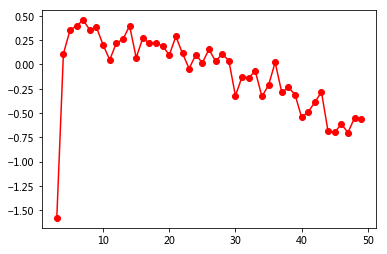In :
data_X_scale = scale(boston.data)
scores = cross_val_score(regr,data_X_scale, boston.target,\
cv = 7)
scores.mean()

Out:
0.45384871359695633

# 使用天涯bbs数据¶

In :
import pandas as pd

df = pd.read_csv('../data/tianya_bbs_threads_list.txt', sep = "\t", header=None)
df=df.rename(columns = {0:'title', 1:'link', 2:'author',3:'author_page', 4:'click', 5:'reply', 6:'time'})
df[:2]

Out:
title link author author_page click reply time
0 【民间语文第161期】宁波px启示:船进港湾人应上岸 /post-free-2849477-1.shtml 贾也 http://www.tianya.cn/50499450 194675 2703 2012-10-29 07:59
1 宁波镇海PX项目引发群体上访 当地政府发布说明(转载) /post-free-2839539-1.shtml 无上卫士ABC http://www.tianya.cn/74341835 88244 1041 2012-10-24 12:41
In :
# 定义这个函数的目的是让读者感受到：
# 抽取不同的样本，得到的结果完全不同。
def randomSplit(dataX, dataY, num):
dataX_train = []
dataX_test = []
dataY_train = []
dataY_test = []
import random
test_index = random.sample(range(len(df)), num)
for k in range(len(dataX)):
if k in test_index:
dataX_test.append([dataX[k]])
dataY_test.append(dataY[k])
else:
dataX_train.append([dataX[k]])
dataY_train.append(dataY[k])
return dataX_train, dataX_test, dataY_train, dataY_test,

In :
import numpy as np

# Use only one feature
data_X = df.reply
# Split the data into training/testing sets
data_X_train, data_X_test, data_y_train, data_y_test = randomSplit(np.log(df.click+1),
np.log(df.reply+1), 20)
# Create linear regression object
regr = linear_model.LinearRegression()
# Train the model using the training sets
regr.fit(data_X_train, data_y_train)
# Explained variance score: 1 is perfect prediction
print('Variance score: %.2f' % regr.score(data_X_test, data_y_test))

Variance score: 0.74

In :
data_X_train

Out:
[[194675, 2703],
[88244, 1041],
[82779, 625],
[45304, 219],
[38132, 835],
[27026, 122],
[24026, 115],
[21497, 378],
[15366, 375],
[8513, 41],
[7191, 61],
[6756, 16],
[6368, 86],
[4990, 0],
[4241, 0],
[3995, 19],
[3720, 2],
[3468, 104],
[3421, 7],
[3233, 70],
[3126, 50],
[2699, 59],
[2456, 2],
[2433, 4],
[2342, 23],
[2257, 142],
[2164, 35],
[2153, 0],
[2151, 35],
[2116, 70],
[2077, 18],
[1981, 24],
[1875, 28],
[1809, 15],
[1795, 1],
[1772, 18],
[1599, 75],
[1516, 44],
[1414, 10],
[1319, 28],
[1306, 36],
[1294, 5],
[1268, 4],
[1219, 3],
[1214, 24],
[1156, 24],
[1154, 16],
[1099, 77],
[1046, 0],
[1033, 6],
[1033, 0],
[998, 35],
[998, 15],
[987, 0],
[947, 4],
[910, 0],
[891, 39],
[852, 0],
[813, 11],
[768, 20],
[746, 7],
[707, 10],
[705, 29],
[702, 18],
[677, 12],
[668, 42],
[667, 0],
[655, 3],
[652, 0],
[624, 9],
[622, 82],
[608, 7],
[601, 16],
[597, 18],
[596, 11],
[584, 0],
[567, 10],
[544, 17],
[531, 7],
[525, 10],
[515, 62],
[508, 12],
[508, 7],
[498, 0],
[496, 1],
[482, 5],
[462, 0],
[458, 41],
[444, 7],
[433, 0],
[421, 1],
[420, 12],
[419, 35],
[410, 8],
[405, 0],
[405, 0],
[400, 14],
[397, 16],
[388, 12],
[381, 7],
[381, 1],
[379, 0],
[362, 1],
[352, 4],
[349, 0],
[348, 8],
[331, 1],
[328, 0],
[327, 6],
[324, 1],
[320, 1],
[315, 0],
[306, 14],
[306, 0],
[300, 0],
[300, 4],
[289, 0],
[288, 1],
[287, 0],
[286, 5],
[278, 3],
[275, 4],
[272, 0],
[269, 1],
[269, 7],
[265, 0],
[261, 0],
[261, 6],
[255, 9],
[252, 7],
[250, 0],
[241, 0],
[235, 5],
[235, 4],
[234, 9],
[232, 7],
[224, 3],
[216, 2],
[214, 24],
[207, 1],
[205, 4],
[197, 4],
[190, 0],
[188, 2],
[187, 0],
[183, 6],
[181, 5],
[176, 0],
[172, 3],
[170, 5],
[170, 0],
[166, 0],
[166, 5],
[165, 0],
[164, 3],
[164, 1],
[161, 0],
[154, 1],
[151, 1],
[151, 2],
[149, 1],
[149, 0],
[149, 3],
[147, 0],
[146, 5],
[145, 0],
[143, 0],
[142, 0],
[139, 5],
[137, 4],
[137, 1],
[136, 0],
[135, 1],
[134, 0],
[133, 1],
[131, 1],
[127, 0],
[125, 0],
[123, 0],
[119, 0],
[118, 0],
[118, 0],
[118, 0],
[116, 0],
[116, 0],
[114, 7],
[113, 0],
[110, 0],
[110, 0],
[109, 0],
[108, 8],
[107, 8],
[106, 0],
[105, 0],
[105, 0],
[105, 10],
[103, 0],
[101, 5],
[100, 6],
[100, 0],
[99, 3],
[99, 1],
[98, 0],
[98, 1],
[98, 1],
[97, 2],
[96, 0],
[96, 0],
[95, 0],
[94, 3],
[93, 0],
[93, 3],
[92, 2],
[90, 1],
[90, 2],
[89, 0],
[88, 3],
[86, 0],
[86, 3],
[85, 0],
[85, 0],
[84, 0],
[84, 1],
[83, 1],
[83, 0],
[82, 0],
[81, 9],
[81, 5],
[81, 2],
[81, 10],
[81, 0],
[80, 0],
[80, 0],
[80, 5],
[78, 0],
[78, 0],
[77, 0],
[76, 3],
[76, 0],
[76, 0],
[75, 0],
[74, 1],
[74, 0],
[73, 0],
[73, 3],
[73, 3],
[73, 0],
[73, 5],
[73, 0],
[73, 0],
[72, 1],
[72, 0],
[64, 2],
[64, 0],
[64, 1],
[64, 0],
[64, 0],
[63, 1],
[62, 3],
[62, 0],
[62, 0],
[61, 1],
[61, 0],
[61, 0],
[61, 0],
[61, 0],
[60, 2],
[60, 3],
[59, 0],
[59, 0],
[59, 0],
[59, 4],
[59, 0],
[59, 0],
[59, 2],
[58, 0],
[58, 0],
[58, 0],
[58, 0],
[57, 1],
[57, 0],
[57, 1],
[57, 4],
[57, 0],
[57, 0],
[56, 0],
[56, 1],
[56, 0],
[56, 0],
[55, 0],
[55, 0],
[54, 0],
[54, 0],
[53, 4],
[53, 0],
[53, 0],
[52, 0],
[52, 0],
[52, 0],
[52, 0],
[52, 1],
[52, 0],
[51, 0],
[51, 0],
[50, 0],
[50, 0],
[50, 1],
[50, 0],
[50, 0],
[50, 0],
[49, 0],
[49, 0],
[49, 0],
[49, 0],
[49, 0],
[48, 0],
[47, 0],
[47, 0],
[47, 0],
[47, 0],
[46, 0],
[46, 0],
[46, 0],
[45, 1],
[45, 1],
[45, 0],
[45, 0],
[44, 0],
[43, 0],
[43, 0],
[43, 0],
[43, 0],
[43, 1],
[43, 1],
[42, 0],
[42, 0],
[42, 1],
[42, 1],
[42, 1],
[42, 2],
[42, 3],
[41, 0],
[41, 0],
[41, 0],
[41, 0],
[40, 0],
[40, 0],
[40, 0],
[40, 1],
[40, 0],
[39, 0],
[39, 0],
[39, 0],
[39, 0],
[39, 1],
[39, 0],
[39, 0],
[38, 1],
[38, 0],
[38, 0],
[38, 0],
[38, 1],
[37, 0],
[37, 0],
[37, 0],
[37, 0],
[36, 0],
[36, 0],
[36, 0],
[36, 0],
[36, 0],
[36, 0],
[36, 1],
[36, 0],
[35, 0],
[35, 0],
[35, 0],
[34, 0],
[34, 2],
[34, 0],
[34, 2],
[34, 0],
[33, 0],
[33, 0],
[33, 0],
[33, 0],
[33, 0],
[33, 0],
[33, 1],
[33, 0],
[33, 0],
[32, 0],
[31, 0],
[31, 0],
[31, 0],
[30, 0],
[30, 0],
[29, 0],
[29, 0],
[29, 0],
[29, 0],
[29, 0],
[29, 0],
[28, 0],
[28, 0],
[28, 0],
[28, 0],
[28, 0],
[27, 0],
[26, 0],
[26, 0],
[26, 0],
[25, 0],
[25, 0],
[25, 0],
[25, 0],
[25, 0],
[24, 0],
[24, 0],
[24, 0],
[24, 0],
[24, 0],
[24, 0],
[23, 0],
[23, 0],
[23, 0],
[23, 0],
[22, 0],
[22, 1],
[21, 0],
[21, 0],
[21, 0],
[20, 0],
[20, 0],
[20, 0],
[19, 0],
[19, 0],
[19, 0],
[17, 0],
[17, 0],
[17, 0],
[17, 0],
[17, 0],
[17, 0],
[15, 0],
[14, 0],
[11, 0]]
In :
y_true, y_pred = data_y_test, regr.predict(data_X_test)

In :
plt.scatter(y_pred, y_true,  color='black')
plt.show()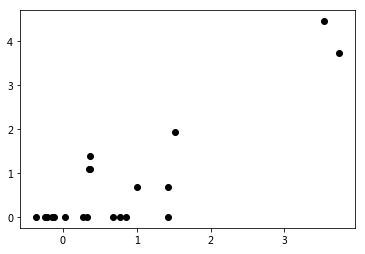In :
# Plot outputs
plt.scatter(data_X_test, data_y_test,  color='black')
plt.plot(data_X_test, regr.predict(data_X_test), color='blue', linewidth=3)
plt.show()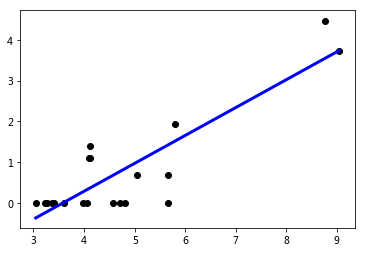In :
# The coefficients
'Coefficients: \n', regr.coef_

Out:
('Coefficients: \n', array([ 0.68334304]))
In :
# The mean square error
"Residual sum of squares: %.2f" % np.mean((regr.predict(data_X_test) - data_y_test) ** 2)

Out:
'Residual sum of squares: 0.40'
In :
df.click_log = [[np.log(df.click[i]+1)] for i in range(len(df))]
df.reply_log = [[np.log(df.reply[i]+1)] for i in range(len(df))]

In :
from sklearn.cross_validation import train_test_split
Xs_train, Xs_test, y_train, y_test = train_test_split(df.click_log, df.reply_log,test_size=0.2, random_state=0)

# Create linear regression object
regr = linear_model.LinearRegression()
# Train the model using the training sets
regr.fit(Xs_train, y_train)
# Explained variance score: 1 is perfect prediction
'Variance score: %.2f' % regr.score(Xs_test, y_test)

Out:
'Variance score: 0.62'
In :
# Plot outputs
plt.scatter(Xs_test, y_test,  color='black')
plt.plot(Xs_test, regr.predict(Xs_test), color='blue', linewidth=3)
plt.show()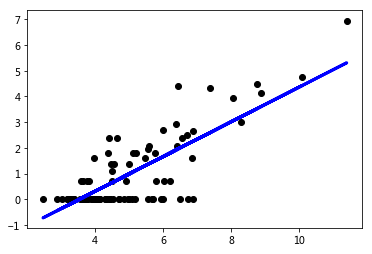In :
from sklearn.cross_validation import cross_val_score

regr = linear_model.LinearRegression()
scores = cross_val_score(regr, df.click_log, \
df.reply_log, cv = 3)
scores.mean()

Out:
-0.68370073919430563
In :
regr = linear_model.LinearRegression()
scores = cross_val_score(regr, df.click_log,
df.reply_log, cv =5)
scores.mean()

Out:
-0.71881497228209845

# 使用sklearn做logistic回归¶

[email protected]

• logistic回归是一个分类算法而不是一个回归算法。
• 可根据已知的一系列因变量估计离散数值（比方说二进制数值 0 或 1 ，是或否，真或假）。
• 简单来说，它通过将数据拟合进一个逻辑函数(logistic function)来预估一个事件出现的概率。
• 因此，它也被叫做逻辑回归。因为它预估的是概率，所以它的输出值大小在 0 和 1 之间（正如所预计的一样）。

$$odds= \frac{p}{1-p} = \frac{probability\: of\: event\: occurrence} {probability \:of \:not\: event\: occurrence}$$

$$ln(odds)= ln(\frac{p}{1-p})$$

$$logit(x) = ln(\frac{p}{1-p}) = b_0+b_1X_1+b_2X_2+b_3X_3....+b_kX_k$$In :
repost = []
for i in df.title:
if u'转载' in i:
repost.append(1)
else:
repost.append(0)

In :
data_X = [[df.click[i], df.reply[i]] for i in range(len(df))]
data_X[:3]

Out:
[[194675, 2703], [88244, 1041], [82779, 625]]
In :
from sklearn.linear_model import LogisticRegression
df['repost'] = repost
model = LogisticRegression()
model.fit(data_X,df.repost)
model.score(data_X,df.repost)

Out:
0.61241970021413272
In :
def randomSplitLogistic(dataX, dataY, num):
dataX_train = []
dataX_test = []
dataY_train = []
dataY_test = []
import random
test_index = random.sample(range(len(df)), num)
for k in range(len(dataX)):
if k in test_index:
dataX_test.append(dataX[k])
dataY_test.append(dataY[k])
else:
dataX_train.append(dataX[k])
dataY_train.append(dataY[k])
return dataX_train, dataX_test, dataY_train, dataY_test,

In :
# Split the data into training/testing sets
data_X_train, data_X_test, data_y_train, data_y_test = randomSplitLogistic(data_X, df.repost, 20)
# Create logistic regression object
log_regr = LogisticRegression()
# Train the model using the training sets
log_regr.fit(data_X_train, data_y_train)
# Explained variance score: 1 is perfect prediction
'Variance score: %.2f' % log_regr.score(data_X_test, data_y_test)

Out:
'Variance score: 0.45'
In :
y_true, y_pred = data_y_test, log_regr.predict(data_X_test)

In :
y_true, y_pred

Out:
([1, 0, 0, 1, 0, 0, 0, 1, 1, 0, 1, 1, 1, 1, 1, 1, 1, 1, 1, 1],
array([0, 0, 1, 1, 1, 1, 1, 1, 1, 1, 1, 1, 1, 1, 1, 1, 1, 1, 1, 1]))
In :
print(classification_report(y_true, y_pred))

             precision    recall  f1-score   support

0       0.50      0.17      0.25         6
1       0.72      0.93      0.81        14

avg / total       0.66      0.70      0.64        20


In :
from sklearn.cross_validation import train_test_split
Xs_train, Xs_test, y_train, y_test = train_test_split(data_X, df.repost, test_size=0.2, random_state=42)

In :
# Create logistic regression object
log_regr = LogisticRegression()
# Train the model using the training sets
log_regr.fit(Xs_train, y_train)
# Explained variance score: 1 is perfect prediction
'Variance score: %.2f' % log_regr.score(Xs_test, y_test)

Out:
'Variance score: 0.60'
In :
print('Logistic score for test set: %f' % log_regr.score(Xs_test, y_test))
print('Logistic score for training set: %f' % log_regr.score(Xs_train, y_train))
y_true, y_pred = y_test, log_regr.predict(Xs_test)
print(classification_report(y_true, y_pred))

Logistic score for test set: 0.595745
Logistic score for training set: 0.613941
precision    recall  f1-score   support

0       1.00      0.03      0.05        39
1       0.59      1.00      0.74        55

avg / total       0.76      0.60      0.46        94


In :
logre = LogisticRegression()
scores = cross_val_score(logre, data_X, df.repost, cv = 3)
scores.mean()

Out:
0.53333333333333333
In :
logre = LogisticRegression()
data_X_scale = scale(data_X)
# The importance of preprocessing in data science and the machine learning pipeline I:
scores = cross_val_score(logre, data_X_scale, df.repost, cv = 3)
scores.mean()

Out:
0.62948717948717947

# 使用sklearn实现贝叶斯预测¶

[email protected]

# Naive Bayes algorithm¶

It is a classification technique based on Bayes’ Theorem with an assumption of independence among predictors.

In simple terms, a Naive Bayes classifier assumes that the presence of a particular feature in a class is unrelated to the presence of any other feature.

why it is known as ‘Naive’? For example, a fruit may be considered to be an apple if it is red, round, and about 3 inches in diameter. Even if these features depend on each other or upon the existence of the other features, all of these properties independently contribute to the probability that this fruit is an apple.

$$p(c|x) = \frac{p(x|c) p(c)}{p(x)}$$

• P(c|x) is the posterior probability of class (c, target) given predictor (x, attributes).
• P(c) is the prior probability of class.
• P(x|c) is the likelihood which is the probability of predictor given class.
• P(x) is the prior probability of predictor.Step 1: Convert the data set into a frequency table

Step 2: Create Likelihood table by finding the probabilities like:

• p(Overcast) = 0.29, p(rainy) = 0.36, p(sunny) = 0.36
• p(playing) = 0.64, p(rest) = 0.36

Step 3: Now, use Naive Bayesian equation to calculate the posterior probability for each class. The class with the highest posterior probability is the outcome of prediction.

## Problem: Players will play if weather is sunny. Is this statement is correct?¶

We can solve it using above discussed method of posterior probability.

$P(Yes | Sunny) = \frac{P( Sunny | Yes) * P(Yes) } {P (Sunny)}$

Here we have P (Sunny |Yes) = 3/9 = 0.33, P(Sunny) = 5/14 = 0.36, P( Yes)= 9/14 = 0.64

Now, $P (Yes | Sunny) = \frac{0.33 * 0.64}{0.36} = 0.60$, which has higher probability.

In :
from sklearn import naive_bayes
'  '.join(dir(naive_bayes))

Out:
'ABCMeta  BaseDiscreteNB  BaseEstimator  BaseNB  BernoulliNB  ClassifierMixin  GaussianNB  LabelBinarizer  MultinomialNB  __all__  __builtins__  __doc__  __file__  __name__  __package__  _check_partial_fit_first_call  abstractmethod  binarize  check_X_y  check_array  check_is_fitted  in1d  issparse  label_binarize  logsumexp  np  safe_sparse_dot  six'
• naive_bayes.GaussianNB Gaussian Naive Bayes (GaussianNB)
• naive_bayes.MultinomialNB([alpha, ...]) Naive Bayes classifier for multinomial models
• naive_bayes.BernoulliNB([alpha, binarize, ...]) Naive Bayes classifier for multivariate Bernoulli models.
In :
#Import Library of Gaussian Naive Bayes model
from sklearn.naive_bayes import GaussianNB
import numpy as np

#assigning predictor and target variables
x= np.array([[-3,7],[1,5], [1,2], [-2,0], [2,3], [-4,0], [-1,1], [1,1], [-2,2], [2,7], [-4,1], [-2,7]])
Y = np.array([3, 3, 3, 3, 4, 3, 3, 4, 3, 4, 4, 4])

In :
#Create a Gaussian Classifier
model = GaussianNB()

# Train the model using the training sets
model.fit(x[:8], Y[:8])

#Predict Output
predicted= model.predict([[1,2],[3,4]])
predicted

Out:
array([4, 3])

# cross-validation¶

k-fold CV, the training set is split into k smaller sets (other approaches are described below, but generally follow the same principles). The following procedure is followed for each of the k “folds”:

• A model is trained using k-1 of the folds as training data;
• the resulting model is validated on the remaining part of the data (i.e., it is used as a test set to compute a performance measure such as accuracy).
In :
data_X_train, data_X_test, data_y_train, data_y_test = randomSplit(df.click, df.reply, 20)
# Train the model using the training sets
model.fit(data_X_train, data_y_train)

#Predict Output
predicted= model.predict(data_X_test)
predicted

Out:
array([41,  2,  0,  0,  0,  0,  0,  0,  0,  0,  0,  0,  0,  0,  0,  0,  0,
0,  0,  0])
In :
model.score(data_X_test, data_y_test)

Out:
0.65000000000000002
In :
from sklearn.cross_validation import cross_val_score

model = GaussianNB()
scores = cross_val_score(model, [[c] for c in df.click],\
df.reply, cv = 7)
scores.mean()

/Users/datalab/Applications/anaconda/lib/python3.5/site-packages/sklearn/cross_validation.py:516: Warning: The least populated class in y has only 1 members, which is too few. The minimum number of labels for any class cannot be less than n_folds=7.
% (min_labels, self.n_folds)), Warning)

Out:
0.53413410073295453

# 决策树¶

• 这个监督式学习算法通常被用于分类问题。
• 它同时适用于分类变量和连续因变量。
• 在这个算法中，我们将总体分成两个或更多的同类群。
• 这是根据最重要的属性或者自变量来分成尽可能不同的组别。## 在上图中你可以看到，根据多种属性，人群被分成了不同的四个小组，来判断 “他们会不会去玩”。¶

### 为了把总体分成不同组别，需要用到许多技术，比如说 Gini、Information Gain、Chi-square、entropy。¶

In :
from sklearn import tree
model = tree.DecisionTreeClassifier(criterion='gini')

In :
data_X_train, data_X_test, data_y_train, data_y_test = randomSplitLogistic(data_X, df.repost, 20)
model.fit(data_X_train,data_y_train)
model.score(data_X_train,data_y_train)

Out:
0.91275167785234901
In :
# Predict
model.predict(data_X_test)

Out:
array([0, 0, 0, 0, 0, 1, 1, 0, 0, 1, 1, 0, 1, 1, 1, 0, 1, 1, 1, 0])
In :
# crossvalidation
scores = cross_val_score(model, data_X, df.repost, cv = 3)
scores.mean()

Out:
0.33461538461538459

# 使用sklearn实现SVM支持向量机¶

[email protected]• 将每个数据在N维空间中用点标出（N是你所有的特征总数），每个特征的值是一个坐标的值。
• 举个例子，如果我们只有身高和头发长度两个特征，我们会在二维空间中标出这两个变量，每个点有两个坐标（这些坐标叫做支持向量）。• 现在，我们会找到将两组不同数据分开的一条直线。
• 两个分组中距离最近的两个点到这条线的距离同时最优化。## 上面示例中的黑线将数据分类优化成两个小组¶

• 两组中距离最近的点（图中A、B点）到达黑线的距离满足最优条件。
• 这条直线就是我们的分割线。接下来，测试数据落到直线的哪一边，我们就将它分到哪一类去。
In :
from sklearn import svm
# Create SVM classification object
model=svm.SVC()

In :
' '.join(dir(svm))

Out:
'LinearSVC LinearSVR NuSVC NuSVR OneClassSVM SVC SVR __all__ __builtins__ __cached__ __doc__ __file__ __loader__ __name__ __package__ __path__ __spec__ base bounds classes l1_min_c liblinear libsvm libsvm_sparse'
In :
data_X_train, data_X_test, data_y_train, data_y_test = randomSplitLogistic(data_X, df.repost, 20)
model.fit(data_X_train,data_y_train)
model.score(data_X_train,data_y_train)

Out:
0.90380313199105144
In :
# Predict
model.predict(data_X_test)

Out:
array([0, 0, 0, 0, 0, 0, 0, 0, 0, 0, 1, 0, 1, 1, 1, 1, 1, 1, 1, 1])
In :
# crossvalidation
scores = []
cvs = [3, 5, 10, 25, 50, 75, 100]
for i in cvs:
score = cross_val_score(model, data_X, df.repost,
cv = i)
scores.append(score.mean() ) # Try to tune cv


In :
plt.plot(cvs, scores, 'b-o')
plt.xlabel('$cv$', fontsize = 20)
plt.ylabel('$Score$', fontsize = 20)
plt.show()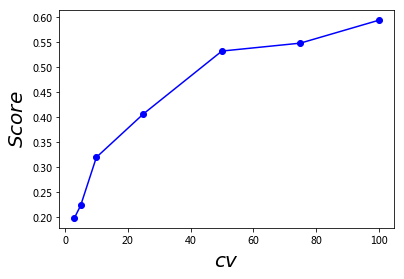# 泰坦尼克号数据分析¶

[email protected]

In :
#Import the Numpy library
import numpy as np
#Import 'tree' from scikit-learn library
from sklearn import tree

In :
import pandas as pd
train = pd.read_csv('../data/tatanic_train.csv', sep = ",")

In :
from sklearn.naive_bayes import GaussianNB

In :
train["Age"] = train["Age"].fillna(train["Age"].median())
train["Fare"] = train["Fare"].fillna(train["Fare"].median())
# x = [[i] for i in train['Age']]
y = train['Age']
y = train['Fare'].astype(int)
#y = [[i] for i in y]

In :
#Create a Gaussian Classifier
model = GaussianNB()

# Train the model using the training sets
nb = model.fit(x[:80], y[:80])
# nb.score(x, y)

In :
help(GaussianNB)

Help on class GaussianNB in module sklearn.naive_bayes:

class GaussianNB(BaseNB)
|  Gaussian Naive Bayes (GaussianNB)
|
|  Can perform online updates to model parameters via partial_fit method.
|  For details on algorithm used to update feature means and variance online,
|  see Stanford CS tech report STAN-CS-79-773 by Chan, Golub, and LeVeque:
|
|      http://i.stanford.edu/pub/cstr/reports/cs/tr/79/773/CS-TR-79-773.pdf
|
|  Read more in the :ref:User Guide <gaussian_naive_bayes>.
|
|  Attributes
|  ----------
|  class_prior_ : array, shape (n_classes,)
|      probability of each class.
|
|  class_count_ : array, shape (n_classes,)
|      number of training samples observed in each class.
|
|  theta_ : array, shape (n_classes, n_features)
|      mean of each feature per class
|
|  sigma_ : array, shape (n_classes, n_features)
|      variance of each feature per class
|
|  Examples
|  --------
|  >>> import numpy as np
|  >>> X = np.array([[-1, -1], [-2, -1], [-3, -2], [1, 1], [2, 1], [3, 2]])
|  >>> Y = np.array([1, 1, 1, 2, 2, 2])
|  >>> from sklearn.naive_bayes import GaussianNB
|  >>> clf = GaussianNB()
|  >>> clf.fit(X, Y)
|  GaussianNB()
|  >>> print(clf.predict([[-0.8, -1]]))
|  
|  >>> clf_pf = GaussianNB()
|  >>> clf_pf.partial_fit(X, Y, np.unique(Y))
|  GaussianNB()
|  >>> print(clf_pf.predict([[-0.8, -1]]))
|  
|
|  Method resolution order:
|      GaussianNB
|      BaseNB
|      abc.NewBase
|      sklearn.base.BaseEstimator
|      sklearn.base.ClassifierMixin
|      builtins.object
|
|  Methods defined here:
|
|  fit(self, X, y, sample_weight=None)
|      Fit Gaussian Naive Bayes according to X, y
|
|      Parameters
|      ----------
|      X : array-like, shape (n_samples, n_features)
|          Training vectors, where n_samples is the number of samples
|          and n_features is the number of features.
|
|      y : array-like, shape (n_samples,)
|          Target values.
|
|      sample_weight : array-like, shape (n_samples,), optional
|          Weights applied to individual samples (1. for unweighted).
|
|          .. versionadded:: 0.17
|             Gaussian Naive Bayes supports fitting with *sample_weight*.
|
|      Returns
|      -------
|      self : object
|          Returns self.
|
|  partial_fit(self, X, y, classes=None, sample_weight=None)
|      Incremental fit on a batch of samples.
|
|      This method is expected to be called several times consecutively
|      on different chunks of a dataset so as to implement out-of-core
|      or online learning.
|
|      This is especially useful when the whole dataset is too big to fit in
|      memory at once.
|
|      This method has some performance and numerical stability overhead,
|      hence it is better to call partial_fit on chunks of data that are
|      as large as possible (as long as fitting in the memory budget) to
|      hide the overhead.
|
|      Parameters
|      ----------
|      X : array-like, shape (n_samples, n_features)
|          Training vectors, where n_samples is the number of samples and
|          n_features is the number of features.
|
|      y : array-like, shape (n_samples,)
|          Target values.
|
|      classes : array-like, shape (n_classes,)
|          List of all the classes that can possibly appear in the y vector.
|
|          Must be provided at the first call to partial_fit, can be omitted
|          in subsequent calls.
|
|      sample_weight : array-like, shape (n_samples,), optional
|          Weights applied to individual samples (1. for unweighted).
|
|          .. versionadded:: 0.17
|
|      Returns
|      -------
|      self : object
|          Returns self.
|
|  ----------------------------------------------------------------------
|  Data and other attributes defined here:
|
|  __abstractmethods__ = frozenset()
|
|  ----------------------------------------------------------------------
|  Methods inherited from BaseNB:
|
|  predict(self, X)
|      Perform classification on an array of test vectors X.
|
|      Parameters
|      ----------
|      X : array-like, shape = [n_samples, n_features]
|
|      Returns
|      -------
|      C : array, shape = [n_samples]
|          Predicted target values for X
|
|  predict_log_proba(self, X)
|      Return log-probability estimates for the test vector X.
|
|      Parameters
|      ----------
|      X : array-like, shape = [n_samples, n_features]
|
|      Returns
|      -------
|      C : array-like, shape = [n_samples, n_classes]
|          Returns the log-probability of the samples for each class in
|          the model. The columns correspond to the classes in sorted
|          order, as they appear in the attribute classes_.
|
|  predict_proba(self, X)
|      Return probability estimates for the test vector X.
|
|      Parameters
|      ----------
|      X : array-like, shape = [n_samples, n_features]
|
|      Returns
|      -------
|      C : array-like, shape = [n_samples, n_classes]
|          Returns the probability of the samples for each class in
|          the model. The columns correspond to the classes in sorted
|          order, as they appear in the attribute classes_.
|
|  ----------------------------------------------------------------------
|  Methods inherited from sklearn.base.BaseEstimator:
|
|  __repr__(self)
|      Return repr(self).
|
|  get_params(self, deep=True)
|      Get parameters for this estimator.
|
|      Parameters
|      ----------
|      deep: boolean, optional
|          If True, will return the parameters for this estimator and
|          contained subobjects that are estimators.
|
|      Returns
|      -------
|      params : mapping of string to any
|          Parameter names mapped to their values.
|
|  set_params(self, **params)
|      Set the parameters of this estimator.
|
|      The method works on simple estimators as well as on nested objects
|      (such as pipelines). The former have parameters of the form
|      <component>__<parameter> so that it's possible to update each
|      component of a nested object.
|
|      Returns
|      -------
|      self
|
|  ----------------------------------------------------------------------
|  Data descriptors inherited from sklearn.base.BaseEstimator:
|
|  __dict__
|      dictionary for instance variables (if defined)
|
|  __weakref__
|      list of weak references to the object (if defined)
|
|  ----------------------------------------------------------------------
|  Methods inherited from sklearn.base.ClassifierMixin:
|
|  score(self, X, y, sample_weight=None)
|      Returns the mean accuracy on the given test data and labels.
|
|      In multi-label classification, this is the subset accuracy
|      which is a harsh metric since you require for each sample that
|      each label set be correctly predicted.
|
|      Parameters
|      ----------
|      X : array-like, shape = (n_samples, n_features)
|          Test samples.
|
|      y : array-like, shape = (n_samples) or (n_samples, n_outputs)
|          True labels for X.
|
|      sample_weight : array-like, shape = [n_samples], optional
|          Sample weights.
|
|      Returns
|      -------
|      score : float
|          Mean accuracy of self.predict(X) wrt. y.


In [ ]:
model.fit(x)

In :
train.head()

Out:
Unnamed: 0 PassengerId Survived Pclass Name Sex Age SibSp Parch Ticket Fare Cabin Embarked
0 0 1 0 3 Braund, Mr. Owen Harris male 22.0 1 0 A/5 21171 7.2500 NaN S
1 1 2 1 1 Cumings, Mrs. John Bradley (Florence Briggs Th... female 38.0 1 0 PC 17599 71.2833 C85 C
2 2 3 1 3 Heikkinen, Miss. Laina female 26.0 0 0 STON/O2. 3101282 7.9250 NaN S
3 3 4 1 1 Futrelle, Mrs. Jacques Heath (Lily May Peel) female 35.0 1 0 113803 53.1000 C123 S
4 4 5 0 3 Allen, Mr. William Henry male 35.0 0 0 373450 8.0500 NaN S
In :
train["Age"] = train["Age"].fillna(train["Age"].median())
#Convert the male and female groups to integer form
train["Sex"][train["Sex"] == "male"] = 0
train["Sex"][train["Sex"] == "female"] = 1

#Impute the Embarked variable
train["Embarked"] = train["Embarked"].fillna('S')
#Convert the Embarked classes to integer form
train["Embarked"][train["Embarked"] == "S"] = 0
train["Embarked"][train["Embarked"] == "C"] = 1
train["Embarked"][train["Embarked"] == "Q"] = 2

/Users/datalab/Applications/anaconda/lib/python3.5/site-packages/ipykernel/__main__.py:3: SettingWithCopyWarning:
A value is trying to be set on a copy of a slice from a DataFrame

See the caveats in the documentation: http://pandas.pydata.org/pandas-docs/stable/indexing.html#indexing-view-versus-copy
app.launch_new_instance()
/Users/datalab/Applications/anaconda/lib/python3.5/site-packages/ipykernel/__main__.py:4: SettingWithCopyWarning:
A value is trying to be set on a copy of a slice from a DataFrame

See the caveats in the documentation: http://pandas.pydata.org/pandas-docs/stable/indexing.html#indexing-view-versus-copy
/Users/datalab/Applications/anaconda/lib/python3.5/site-packages/ipykernel/__main__.py:9: SettingWithCopyWarning:
A value is trying to be set on a copy of a slice from a DataFrame

See the caveats in the documentation: http://pandas.pydata.org/pandas-docs/stable/indexing.html#indexing-view-versus-copy
/Users/datalab/Applications/anaconda/lib/python3.5/site-packages/ipykernel/__main__.py:10: SettingWithCopyWarning:
A value is trying to be set on a copy of a slice from a DataFrame

See the caveats in the documentation: http://pandas.pydata.org/pandas-docs/stable/indexing.html#indexing-view-versus-copy
/Users/datalab/Applications/anaconda/lib/python3.5/site-packages/ipykernel/__main__.py:11: SettingWithCopyWarning:
A value is trying to be set on a copy of a slice from a DataFrame

See the caveats in the documentation: http://pandas.pydata.org/pandas-docs/stable/indexing.html#indexing-view-versus-copy

In :
#Create the target and features numpy arrays: target, features_one
target = train['Survived'].values
features_one = train[["Pclass", "Sex", "Age", "Fare"]].values

#Fit your first decision tree: my_tree_one
my_tree_one = tree.DecisionTreeClassifier()
my_tree_one = my_tree_one.fit(features_one, target)
#Look at the importance of the included features and print the score
print(my_tree_one.feature_importances_)
print(my_tree_one.score(features_one, target))

[ 0.13031677  0.31274009  0.23443048  0.32251266]
0.977553310887

In :
test = pd.read_csv('../data/tatanic_test.csv', sep = ",")
# Impute the missing value with the median
test.Fare = test.Fare.median()
test["Age"] = test["Age"].fillna(test["Age"].median())
#Convert the male and female groups to integer form
test["Sex"][test["Sex"] == "male"] = 0
test["Sex"][test["Sex"] == "female"] = 1

#Impute the Embarked variable
test["Embarked"] = test["Embarked"].fillna('S')
#Convert the Embarked classes to integer form
test["Embarked"][test["Embarked"] == "S"] = 0
test["Embarked"][test["Embarked"] == "C"] = 1
test["Embarked"][test["Embarked"] == "Q"] = 2

# Extract the features from the test set: Pclass, Sex, Age, and Fare.
test_features = test[["Pclass","Sex", "Age", "Fare"]].values

# Make your prediction using the test set
my_prediction = my_tree_one.predict(test_features)

# Create a data frame with two columns: PassengerId & Survived. Survived contains your predictions
PassengerId =np.array(test['PassengerId']).astype(int)
my_solution = pd.DataFrame(my_prediction, PassengerId, columns = ["Survived"])

/Users/datalab/Applications/anaconda/lib/python3.5/site-packages/ipykernel/__main__.py:3: SettingWithCopyWarning:
A value is trying to be set on a copy of a slice from a DataFrame

See the caveats in the documentation: http://pandas.pydata.org/pandas-docs/stable/indexing.html#indexing-view-versus-copy
app.launch_new_instance()
/Users/datalab/Applications/anaconda/lib/python3.5/site-packages/ipykernel/__main__.py:6: SettingWithCopyWarning:
A value is trying to be set on a copy of a slice from a DataFrame

See the caveats in the documentation: http://pandas.pydata.org/pandas-docs/stable/indexing.html#indexing-view-versus-copy
/Users/datalab/Applications/anaconda/lib/python3.5/site-packages/ipykernel/__main__.py:7: SettingWithCopyWarning:
A value is trying to be set on a copy of a slice from a DataFrame

See the caveats in the documentation: http://pandas.pydata.org/pandas-docs/stable/indexing.html#indexing-view-versus-copy
/Users/datalab/Applications/anaconda/lib/python3.5/site-packages/ipykernel/__main__.py:12: SettingWithCopyWarning:
A value is trying to be set on a copy of a slice from a DataFrame

See the caveats in the documentation: http://pandas.pydata.org/pandas-docs/stable/indexing.html#indexing-view-versus-copy
/Users/datalab/Applications/anaconda/lib/python3.5/site-packages/ipykernel/__main__.py:13: SettingWithCopyWarning:
A value is trying to be set on a copy of a slice from a DataFrame

See the caveats in the documentation: http://pandas.pydata.org/pandas-docs/stable/indexing.html#indexing-view-versus-copy
/Users/datalab/Applications/anaconda/lib/python3.5/site-packages/ipykernel/__main__.py:14: SettingWithCopyWarning:
A value is trying to be set on a copy of a slice from a DataFrame

See the caveats in the documentation: http://pandas.pydata.org/pandas-docs/stable/indexing.html#indexing-view-versus-copy

In :
my_solution[:3]

Out:
Survived
892 0
893 0
894 1
In :
# Check that your data frame has 418 entries
my_solution.shape

Out:
(418, 1)
In :
# Write your solution to a csv file with the name my_solution.csv
my_solution.to_csv("../data/tatanic_solution_one.csv", index_label = ["PassengerId"])

In :
# Create a new array with the added features: features_two
features_two = train[["Pclass","Age","Sex","Fare",\
"SibSp", "Parch", "Embarked"]].values

#Control overfitting by setting "max_depth" to 10 and "min_samples_split" to 5 : my_tree_two
max_depth = 10
min_samples_split = 5
my_tree_two = tree.DecisionTreeClassifier(max_depth = max_depth,
min_samples_split = min_samples_split,
random_state = 1)
my_tree_two = my_tree_two.fit(features_two, target)

#Print the score of the new decison tree
print(my_tree_two.score(features_two, target))

0.905723905724

In :
# create a new train set with the new variable
train_two = train
train_two['family_size'] = train.SibSp + train.Parch + 1

# Create a new decision tree my_tree_three
features_three = train[["Pclass", "Sex", "Age", \
"Fare", "SibSp", "Parch", "family_size"]].values

my_tree_three = tree.DecisionTreeClassifier()
my_tree_three = my_tree_three.fit(features_three, target)

# Print the score of this decision tree
print(my_tree_three.score(features_three, target))

0.979797979798

In :
#Import the RandomForestClassifier
from sklearn.ensemble import RandomForestClassifier

#We want the Pclass, Age, Sex, Fare,SibSp, Parch, and Embarked variables
features_forest = train[["Pclass", "Age", "Sex", "Fare", "SibSp", "Parch", "Embarked"]].values

#Building the Forest: my_forest
n_estimators = 100
forest = RandomForestClassifier(max_depth = 10, min_samples_split=2,
n_estimators = n_estimators, random_state = 1)
my_forest = forest.fit(features_forest, target)

#Print the score of the random forest
print(my_forest.score(features_forest, target))

#Compute predictions and print the length of the prediction vector:test_features, pred_forest
test_features = test[["Pclass", "Age", "Sex", "Fare", "SibSp", "Parch", "Embarked"]].values
pred_forest = my_forest.predict(test_features)
print(len(test_features))
print(pred_forest[:3])

0.939393939394
418
[0 0 0]

In :
#Request and print the .feature_importances_ attribute
print(my_tree_two.feature_importances_)
print(my_forest.feature_importances_)

#Compute and print the mean accuracy score for both models
print(my_tree_two.score(features_two, target))
print(my_forest.score(features_two, target))

[ 0.14130255  0.17906027  0.41616727  0.17938711  0.05039699  0.01923751
0.0144483 ]
[ 0.10384741  0.20139027  0.31989322  0.24602858  0.05272693  0.04159232
0.03452128]
0.905723905724
0.939393939394


# 阅读材料¶

The "Python Machine Learning" book code repository and info resource https://github.com/rasbt/python-machine-learning-book

An Introduction to Statistical Learning (James, Witten, Hastie, Tibshirani, 2013) : Python code https://github.com/JWarmenhoven/ISLR-python

BuildingMachineLearningSystemsWithPython https://github.com/luispedro/BuildingMachineLearningSystemsWithPython